## 凯迪拉克新战略解析 品牌独立/更多新车

2014年11月03日 16:24 来源：www.chextx.com 超过：次关注 阅读全文

作为与好莱坞电影一起成长起来的美国文化符号，凯迪拉克并没有像好莱坞大片一样一直站在时代的最前沿，在梦想与现实中的苦苦挣扎，凯迪拉克一直在矛盾中徐徐前进。在整个2013年中，凯迪拉克完成全球25.1万辆销量，虽然同比增长近三成，但是在通用2013年971万辆的总销量中，这个数量仅仅是汪洋中的一朵浪花。当然，通用汽车显然并不满足于这样的现状，作为其旗下的唯一豪华车品牌，一场凯迪拉克力求快速复兴的“大戏”即将拉开序幕，而我将其总结为三个关键词，“独立”、“新命名”、“查漏补缺”。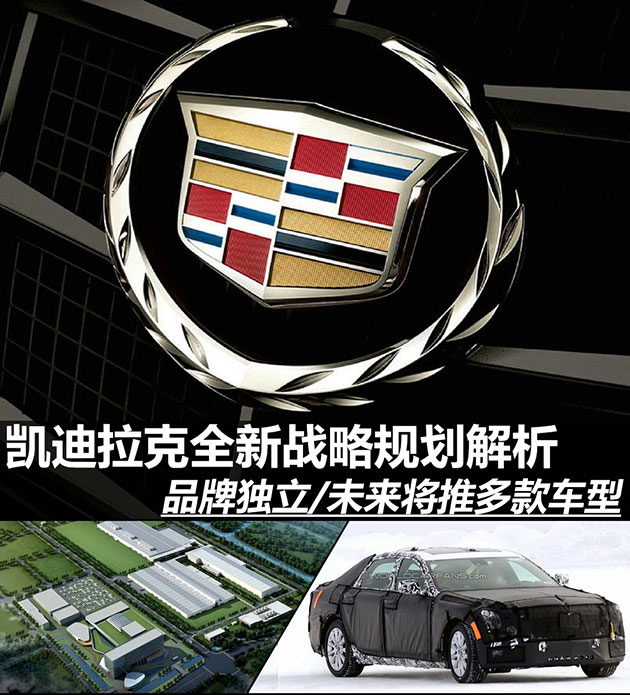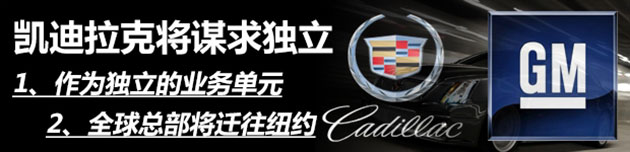在今年9月份，通用公布了全球2014年至2018年中期战略规划，其中凯迪拉克被特别“点名”，未来将会成为一个独立的业务单元。这意味着在包括品牌、市场、规划以及产品定位等方面，凯迪拉克都将拥有决定权。而其全球总部也将迁往纽约，但产品技术研发团队和相关生产制造部门则会留在密歇根州不受影响，这个计划将会在2015年第二季度正式实施。

凯迪拉克品牌新任总裁Johan de Nysschen曾表示：“凯迪拉克要重归豪华车第一线首先要做的就是与通用汽车划清界限，建立起独有的品牌属性和品牌文化。凯迪拉克的任务是在全球豪华品牌阵营中重塑卓越的地位。这项充满挑战和突破性的任务需要一个鲜明且专注的新机构；凯迪拉克将不仅是一个部门或者一个品牌，而将是通用汽车精粹的集中体现。”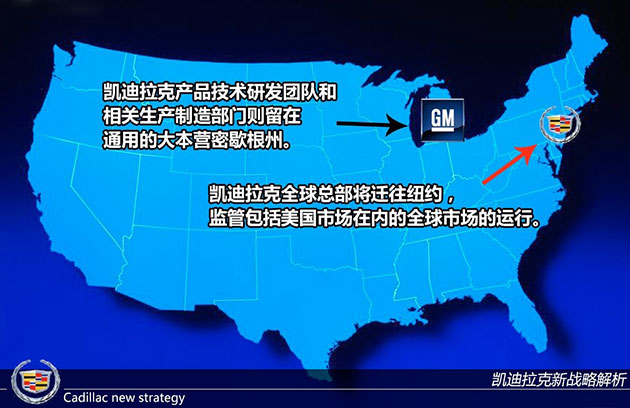新计划实施后，凯迪拉克新总部坐镇世界经济的中心纽约，将更有利于监管包括美国市场在内的全球市场的运行，其在营销和销售角度将拥有更加独立的地位，对于品牌定位、市场的把握以及产品的规划也更具有决定权。对于通用而言，其与竞争对手大众等相比，缺乏的正是高端品牌的布局，所以凯迪拉克的独立若能够取得很好的效果，对于通用整体布局而言是十分有利的。随着新战略计划的公布，凯迪拉克顺势还公布了全新的车型命名体系以及未来车型规划。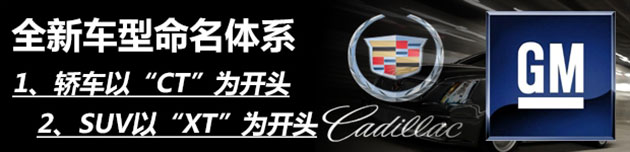说起凯迪拉克全新的车型命名体系，我们必须谈谈凯迪拉克新任总裁Johan de Nysschen。他曾历任奥迪美国总裁、日产旗下英菲尼迪品牌总裁等职务，在其于英菲尼迪任职期间，正是由他操刀对英菲尼迪的车型体系进行了重新梳理。转战凯迪拉克后，Johan de Nysschen显然将其在英菲尼迪所用的招式套用在凯迪拉克上。

未来，凯迪拉克将取消现有的车型命名方式，采用字母组合+数字的方式命名。所有的轿车型号都将采用英文字母组合“CT”为开头，所有SUV车型将采用“XT”为开头，字母后的数字将反映车型相应的尺寸，以及在凯迪拉克产品型谱中的定位。采用全新命名的第一款车为凯迪拉克目前正在研发的全新豪华车，其被命名为CT6。不过从市场角度考虑，更名将是一个循序渐进的过程，凯迪拉克计划将只在推出改款或全新车型时，才会采用新的命名规则，所以ATS、CTS和XTS等车型的命名在未来一段时间内将不会发生变化。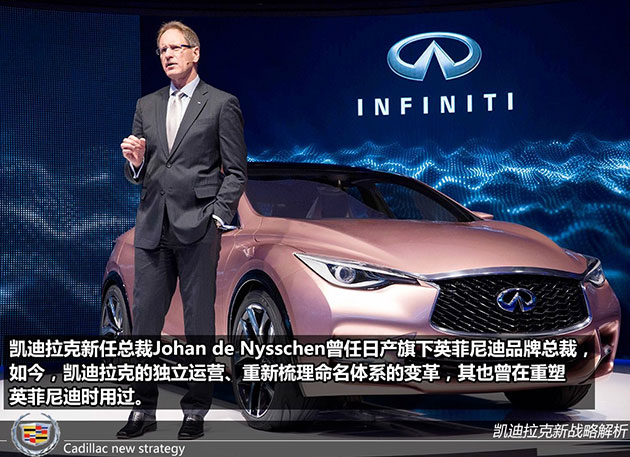未来凯迪拉克所有车型统一采用简化的命名方式之后，将有利于提高其旗下车型的辨识度，更加便于产品向消费者进行推广。另外，现有的命名方式相对较为混乱，对于产品的规划等并没有太多益处，新的命名方式有利于对产品线进行修正，同时进一步扩展产品线范围。不过，改变车型的命名可能会在初期引起市场对于凯迪拉克产品的困惑，而且如何让消费者快速接受新的命名也是一个必须要面对的问题。

1 2 3

#### 相关文章

﻿
• 快速找车
• 选择品牌
• 选择品牌
• A  奥迪
• A  阿斯顿·马丁
• A  阿尔法·罗密欧
• B  宝沃
• B  布加迪
• B  巴博斯
• B  保时捷
• B  宾利
• B  奔驰
• B  宝马
• B  本田
• B  别克
• B  标致
• B  比亚迪
• B  宝骏
• B  北汽制造
• B  北汽新能源
• B  北汽幻速
• B  北汽威旺
• B  北京汽车
• B  奔腾
• B  北汽绅宝
• B  北汽昌河
• C  长安欧尚
• C  长安
• C  长安凯程
• C  长城
• D  大众
• D  道奇
• D  DS
• D  东南
• D  东风风神
• D  东风风行
• D  东风小康
• D  东风风度
• D  东风
• F  福特
• F  丰田
• F  菲亚特
• F  法拉利
• F  福田
• F  福迪
• F  福汽启腾
• G  观致
• G  广汽传祺
• G  广汽吉奥
• G  GMC
• H  红旗
• H  汉腾汽车
• H  哈弗
• H  哈飞
• H  海格
• H  海马
• H  华颂
• H  黄海
• H  华泰
• H  恒天
• J  几何汽车
• J  捷达
• J  吉利汽车
• J  捷豹
• J  Jeep
• J  江淮
• J  江铃
• J  金杯
• J  九龙
• J  金旅
• K  凯翼
• K  凯迪拉克
• K  克莱斯勒
• K  科尼塞克
• K  卡威
• K  开瑞
• L  路虎
• L  林肯
• L  劳斯莱斯
• L  兰博基尼
• L  雷克萨斯
• L  铃木
• L  领克
• L  雷诺
• L  理念
• L  力帆
• L  莲花汽车
• L  猎豹
• L  路特斯
• L  陆风
• M  马自达
• M  MG
• M  MINI
• M  玛莎拉蒂
• M  摩根
• M  迈凯轮
• N  纳智捷
• O  欧拉
• O  欧宝
• O  讴歌
• O  欧朗
• Q  奇瑞
• Q  起亚
• Q  启辰
• R  日产
• R  荣威
• R  瑞麒
• S  SERES赛力斯
• S  三菱
• S  斯威汽车
• S  萨博
• S  smart
• S  斯柯达
• S  斯巴鲁
• S  思铭
• S  双龙
• S  上汽大通
• S  双环
• T  特斯拉
• T  腾势
• W  蔚来
• W  沃尔沃
• W  WEY
• W  五菱汽车
• W  五十铃
• W  威兹曼
• W  威麟
• X  现代
• X  雪佛兰
• X  星途
• X  雪铁龙
• X  小鹏汽车
• X  西雅特
• Y  一汽
• Y  英菲尼迪
• Y  英致
• Y  依维柯
• Y  野马汽车
• Y  永源
• Z  众泰
• Z  中华
• Z  中兴
• Z  知豆
• 选择车系
• 选择车系
• 车型对比
• 选择品牌
• 选择品牌
• A  奥迪
• A  阿斯顿·马丁
• A  阿尔法·罗密欧
• B  宝沃
• B  布加迪
• B  巴博斯
• B  保时捷
• B  宾利
• B  奔驰
• B  宝马
• B  本田
• B  别克
• B  标致
• B  比亚迪
• B  宝骏
• B  北汽制造
• B  北汽新能源
• B  北汽幻速
• B  北汽威旺
• B  北京汽车
• B  奔腾
• B  北汽绅宝
• B  北汽昌河
• C  长安欧尚
• C  长安
• C  长安凯程
• C  长城
• D  大众
• D  道奇
• D  DS
• D  东南
• D  东风风神
• D  东风风行
• D  东风小康
• D  东风风度
• D  东风
• F  福特
• F  丰田
• F  菲亚特
• F  法拉利
• F  福田
• F  福迪
• F  福汽启腾
• G  观致
• G  广汽传祺
• G  广汽吉奥
• G  GMC
• H  红旗
• H  汉腾汽车
• H  哈弗
• H  哈飞
• H  海格
• H  海马
• H  华颂
• H  黄海
• H  华泰
• H  恒天
• J  几何汽车
• J  捷达
• J  吉利汽车
• J  捷豹
• J  Jeep
• J  江淮
• J  江铃
• J  金杯
• J  九龙
• J  金旅
• K  凯翼
• K  凯迪拉克
• K  克莱斯勒
• K  科尼塞克
• K  卡威
• K  开瑞
• L  路虎
• L  林肯
• L  劳斯莱斯
• L  兰博基尼
• L  雷克萨斯
• L  铃木
• L  领克
• L  雷诺
• L  理念
• L  力帆
• L  莲花汽车
• L  猎豹
• L  路特斯
• L  陆风
• M  马自达
• M  MG
• M  MINI
• M  玛莎拉蒂
• M  摩根
• M  迈凯轮
• N  纳智捷
• O  欧拉
• O  欧宝
• O  讴歌
• O  欧朗
• Q  奇瑞
• Q  起亚
• Q  启辰
• R  日产
• R  荣威
• R  瑞麒
• S  SERES赛力斯
• S  三菱
• S  斯威汽车
• S  萨博
• S  smart
• S  斯柯达
• S  斯巴鲁
• S  思铭
• S  双龙
• S  上汽大通
• S  双环
• T  特斯拉
• T  腾势
• W  蔚来
• W  沃尔沃
• W  WEY
• W  五菱汽车
• W  五十铃
• W  威兹曼
• W  威麟
• X  现代
• X  雪佛兰
• X  星途
• X  雪铁龙
• X  小鹏汽车
• X  西雅特
• Y  一汽
• Y  英菲尼迪
• Y  英致
• Y  依维柯
• Y  野马汽车
• Y  永源
• Z  众泰
• Z  中华
• Z  中兴
• Z  知豆
• 选择车系
• 选择车系
• 选择车型
• 选择车型
• 意见反馈